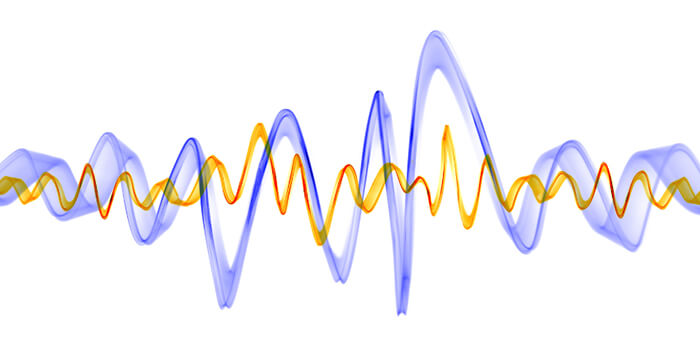# Bands and frequencies – common designationsRadio waves are electromagnetic oscillations that propagate in space at the speed of light. Electromagnetic radiation is characterized by frequency, wavelength and power of the energy carried. The frequency of electromagnetic waves shows how many times per second the direction of the electric current changes in the emitter and therefore how many times per second the value of the electric and magnetic fields change in each point of space.

Frequency is measured in hertz (Hz). 1 Hz is one oscillation per second, 1 megahertz (MHz) is a million oscillations per second. Knowing that the speed of electromagnetic waves is equal to the speed of light, we can determine the distance between points in space where the electric (or magnetic) field is in the same phase. This distance is called the wavelength. The radio waves (radio frequencies) used in radio engineering occupy a region, or more scientifically, a spectrum from 10,000 m (30 kHz) to 0.1 mm (3,000 GHz). International agreements divide the entire spectrum of radio waves used in radio communications into bands:

In addition to separating the frequency range on the basis of wavelength, the following designations are used in mobile official and civil communications:

Generic selectors
Exact matches only
Search in title
Search in content
Post Type Selectors